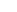# Aapo Hyvärinen## Code for Orthogonal Connectivity Factorization

A. Hyvärinen, J. Hirayama, V. Kiviniemi, and M. Kawanabe. Orthogonal Connectivity Factorization: Interpretable Decomposition of Variability in Correlation Matrices Neural Computation, in press.
(An earlier version, called dynamic connectivity factorization, was published in Proceedings of PRNI2014. The methods are essentially the same, we just changed the name to emphasize the generality of the method.)

The method takes as input a set of connectivity matrices (or network connection matrices, or weighted graph adjacency matrices). The connectivity matrices can be computed for

• Different time windows, to analyse nonstationarity of a multivariate time series (dynamic connectivity), or
• Different subjects, to analyse interindividual differences, or
• Different experimental conditions, to analyse the effect of experimental manipulations, etc.
The connectivity matrices are assumed to be symmetric (at least in the basic version of the method), typically they would be correlations. This matlab implementation is not designed to handle very high-dimensional matrices (e.g. raw voxels in fMRI), so for fMRI you should reduce the dimension to a few hundred ROI's before using this code.

Collect the connectivity matrices in a tensor so that connTensor(:,:,i) contains the i-th matrix. The basic call of the code is then

`[W,V]=ocf(connTensor);`
Here, the first rows of W and V, i.e. W(1,:), V(1,:) gives one component pair of maximum connectivity variability. That is, the connectivity between the components (spatial patterns) given in the data space by those two rows explain more of the connectivity differences than any other pair of linear components.

Just like in PCA, the method gives you more components than one, so that the k-th rows W(k,:) and V(k,:) gives you the k-th component pair. So, the rows of W and V should be interpreted as patterns in the data space. They should always be interpreted in pairs, since it is the connectivity between those two components that changes maximally.

See the ocf.m file for different additional options. For example, the default number of components computed (five) can be changed by just giving the number as an additional parameter like in ocf(connTensor,20).

While some parts of the papers above were written for the purpose of brain imaging, there is absolutely no need for the data to be from brain imaging. If you just have a number of correlation or covariance matrices, you can input them in to the method, and it will give you linear components in the data space such that their correlations changes as much as possible over the correlations matrices.

Page updated in Oct 2015 by Aapo Hyvärinen.